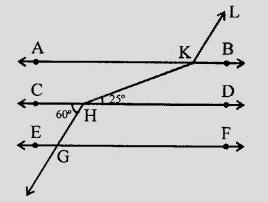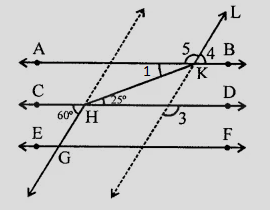"
">

# In the figure, $AB \parallel CD \parallel EF$ and $GH \parallel KL$. Find $\angle HKL$."

Given:

$AB \parallel CD \parallel EF$ and $GH \parallel KL$.

To do:

We have to find $\angle HKL$.

Solution:

Produce $LK$ and $GH$$AB \parallel CD$ and $HK$ is the transversal.

This implies,

$\angle 1 = 25^o$                (Alternate angles)

$\angle 3 = 60^o$                  (Corresponding angles)

$\angle 4 = \angle 3 = 60^o$                  (Corresponding angles)

$\angle 4 + \angle 5 = 180^o$                  (Linear pair)

$60^o + \angle 5 = 180^o$

$\angle 5 = 180^o - 60^o$

$\angle 5 = 120^o$

Therefore,

$\angle HKL = \angle 1 + \angle 5$

$= 25^o + 120^o$

$= 145^o$

Hence, $\angle HKL = 145^o$.

Updated on: 10-Oct-2022

61 Views